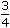# Aptitude - Time and Work

### Exercise :: Time and Work - General Questions

26.

A and B can do a work in 8 days, B and C can do the same work in 12 days. A, B and C together can finish it in 6 days. A and C together will do it in :

 A. 4 days B. 6 days C. 8 days D. 12 days

Explanation:

 (A + B + C)'s 1 day's work = 1 ; 6

 (A + B)'s 1 day's work = 1 ; 8

 (B + C)'s 1 day's work = 1 . 12(A + C)'s 1 day's work
 =2 x 1-1 + 16 8 12
 =1 - 53 24
 = 3 24
 = 1 . 8

So, A and C together will do the work in 8 days.

27.

A can finish a work in 24 days, B in 9 days and C in 12 days. B and C start the work but are forced to leave after 3 days. The remaining work was done by A in:

A. 5 days
B. 6 days
C. 10 days
D.
 10 1 days 2

Explanation:

 (B + C)'s 1 day's work =1 + 1= 7 . 9 12 36

 Work done by B and C in 3 days =7 x 3= 7 . 36 12

 Remaining work =1 - 7= 5 . 12 12

 Now, 1 work is done by A in 1 day. 24

 So, 5 work is done by A in24 x 5= 10 days. 12 12

28.

X can do a piece of work in 40 days. He works at it for 8 days and then Y finished it in 16 days. How long will they together take to complete the work?

A.

 13 1 days 3

B. 15 days
C. 20 days
D. 26 days

Explanation:

 Work done by X in 8 days =1 x 8= 1 . 40 5

 Remaining work =1 - 1= 4 . 5 5

 Now, 4 work is done by Y in 16 days. 5

 Whole work will be done by Y in16 x 5= 20 days. 4X's 1 day's work = 1 , Y's 1 day's work = 1 . 40 20

 (X + Y)'s 1 day's work =1 + 1= 3 . 40 20 40

 Hence, X and Y will together complete the work in40= 13 1 days. 3 3

29.

A and B can do a job together in 7 days. A is 1times as efficient as B. The same job can be done by A alone in :

A.

 9 1 days 3

B. 11 days
C.

 12 1 days 4

D.

 16 1 days 3

Explanation:

 (A's 1 day's work) : (B's 1 day's work) = 7 : 1   =   7 : 4. 4

Let A's and B's 1 day's work be 7x and 4x respectively.

 Then, 7x + 4x = 111x = 1x = 1 . 7 7 77A's 1 day's work =1 x 7= 1 . 77 11

30.

A and B together can do a piece of work in 30 days. A having worked for 16 days, B finishes the remaining work alone in 44 days. In how many days shall B finish the whole work alone?

 A. 30 days B. 40 days C. 60 days D. 70 days

Explanation:

Let A's 1 day's work = x and B's 1 day's work = y.

 Then, x + y = 1 and 16x + 44y = 1. 30

 Solving these two equations, we get: x = 1 and y = 1 60 60B's 1 day's work = 1 . 60

Hence, B alone shall finish the whole work in 60 days.